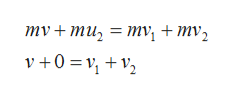# The eight ball, which has a mass of m = 0.5 kg, is initially moving with a velocity v = 4.7i m/s. It strikes the six ball inelastically, which has an identical mass and is initially at rest. After the collision the eight ball is deflected by an angle of θ = 19° and the six ball is deflected by an angle of Φ = 13°, as shown in the figure. a. Write an expression for the magnitude of six ball's velocity, in terms of the angles given in the problem and the magnitude of the eight ball's initial velocity, v. (b)  What is the magnitude of the velocity, in meters per second, of the six ball? (c)  What is the magnitude of the velocity of the eight ball, in meters per second, after the collision?

Question
150 views

The eight ball, which has a mass of m = 0.5 kg, is initially moving with a velocity v = 4.7i m/s. It strikes the six ball inelastically, which has an identical mass and is initially at rest. After the collision the eight ball is deflected by an angle of θ = 19° and the six ball is deflected by an angle of Φ = 13°, as shown in the figure.

a. Write an expression for the magnitude of six ball's velocity, in terms of the angles given in the problem and the magnitude of the eight ball's initial velocity, v.

(b)  What is the magnitude of the velocity, in meters per second, of the six ball?

(c)  What is the magnitude of the velocity of the eight ball, in meters per second, after the collision?

check_circle

Step 1

Given:

Mass of the eight ball, m = 0.5 kg

Initial velocity of the eight ball, v = 4.7i m/s

Mass of the sixth ball, m = 0.5 kg

Initial velocity of the sixth ball, u2 = 0

Angle of deflection of eighth ball = 19ο

Angle of deflection of sixth ball = 13ο

Final velocity of the eight ball = v1

Final velocity of the sixth ball = v2

Step 2

Part (a): on applying the law of the conservation of momentumhelp_outlineImage Transcriptioncloseту, + my, my+ mu, 3D тv, + mv, v +0 2 = 1 fullscreen
Step 3

On applying the conservation of ...

### Want to see the full answer?

See Solution

#### Want to see this answer and more?

Solutions are written by subject experts who are available 24/7. Questions are typically answered within 1 hour.*

See Solution
*Response times may vary by subject and question.
Tagged in

### Work,Power and Energy